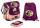# Trees - orchard

The 3/5 trees are apple trees and 1/3 of the trees are cherries. The remaining 5 trees are pear trees. How many trees are in the orchard.

Result

n =  75

#### Solution:

3/5n + 1/3n + 5 = n

n = 75

n = 75

Calculated by our simple equation calculator.

Leave us a comment of example and its solution (i.e. if it is still somewhat unclear...):

Showing 0 comments:Be the first to comment!#### To solve this example are needed these knowledge from mathematics:

Need help calculate sum, simplify or multiply fractions? Try our fraction calculator. Do you have a linear equation or system of equations and looking for its solution? Or do you have quadratic equation?

## Next similar examples:

1. Spain vs USASpain lost to the US by 4 goals. In the match total fell 10 goals. How many goals gave the Spain and how the United States?
2. Boys and girlsThere are 48 children in the sports club, boys are 10 more than girls. How many girls go to the club?
3. ClassroomThere are eighty more girls in the class than boys. Boys are 40 percent and girls are 60 percent. How many are boys and how many girls?
4. Rabbits 3Viju has 40 chickens and rabbits. If in all there are 90 legs. How many rabbits are there with Viju?
5. BarbaraBarborka goes to school with backpack that was 2 - times more expensive than a bag slipper. If backpack was 36 euros cheaper it was cost same as bag slipper. How many cost backpack and how many bas slipper?
6. Football match 4In a football match with the Italy lost 3 goals with Germans. Totally fell 5 goals in the match. Determine the number of goals of Italy and Germany.
7. Simple equation 6Solve equation with one variable: X/2+X/3+X/4=X+4
8. Two numbersThe sum of the two numbers is 1. Find both numbers if you know that half of the first is equal to one seventh of the second number.
9. PC disksPeter has 45 disks in three colors. One-fifth of the disks are blue, red are twice more than the white. How much is blue, red and white disks?
10. Value 4If 5/18=425 what is the value 13/18?
11. Equation with xSolve the following equation: 2x- (8x + 1) - (x + 2) / 5 = 9
12. Father and sonWhen I was 11, my father was 35 years old. Today, my father has three times more years than me. How old is she?
13. Substitutionsolve equations by substitution: x+y= 11 y=5x-25
14. FractionsThree-quarters of an unknown number are 4/5. What is 5/6 of this unknown number?
15. Equation with fractionsSolve equation: ? It is equation with fractions.
16. BlackberriesDaniel, Jolana and Stano collected together 34 blackberries. Daniel collected 8 blackberries more than Jolana, Jolana 4 more than Stano. Determine the number blackberries each collected .
17. Linear systemSolve this linear system (two linear equations with two unknowns): x+y =36 19x+22y=720